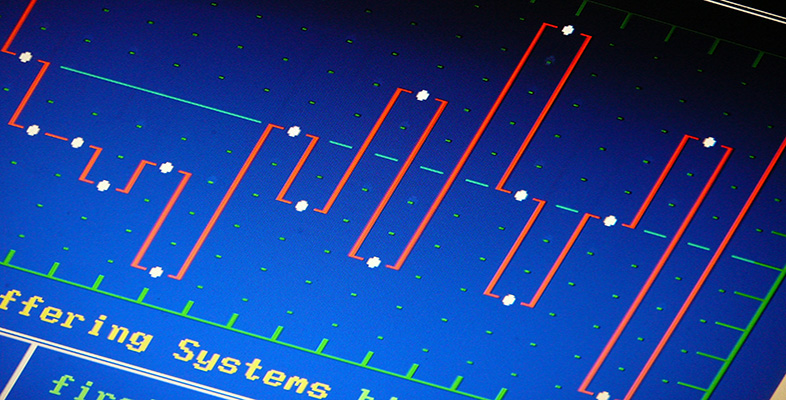Science, Maths & Technology

### Become an OU studentInterpreting data: Boxplots and tables

Start this free course now. Just create an account and sign in. Enrol and complete the course for a free statement of participation or digital badge if available.

# 3.4 Guidelines for graphics

1. Data in the form of counts of individual entities (for example, people, animals, power stations) in a small set of discrete categories can be presented in bar charts or pie charts. For most purposes, bar charts are preferable. Pie charts draw particular attention to the proportions in which the entities are split between the different categories. However, they do so by representing the proportions by angles, and even when the main interest lies in the proportions, bar charts may well be easier to ‘read’.

2. For data in the form of category counts where the interest lies in comparing two or more data sets (as in Example 2.3 of Unit A1 ), it can be useful to produce a bar chart where the corresponding bars for the different data sets are plotted side by side.

3. To examine the pattern of distribution of values of a continuous (measured) variable, a histogram is an appropriate graphic. An alternative is a boxplot. However, neither sort of plot gives direct information about the number of observations in the data set, and it can be risky to draw firm conclusions about the pattern of distribution when the number of observations is small.

4. Boxplots give a simple representation of the values of a continuous variable. They can also be used for discrete variables where the categories are numbers (such as the counts of family sizes in Figure 1.7. A boxplot shows less detail about a distribution than an appropriate histogram or bar chart, but the amount of detail in a boxplot is often sufficient.

5. Boxplots are particularly useful for comparing two or more data sets, because the corresponding boxplots can be drawn against a common scale on the same diagram. (It is difficult to do this clearly with more than one histogram.)

6. Scatterplots are used when the values of two numerical variables have been obtained from each of a number of individual entities. The aim of the plot is to investigate the relationship between the values of the two variables.

7. Line plots can be useful, particularly for time series data, because they draw attention to the way that one or more variables have changed over time.

8. In some circumstances, particularly when the data are very skew, more informative boxplot or scatterplot can be produced by transforming the data first.

This section ends with a more substantial activity. This will provide you with some further experience of dealing with data in tables. You will also be asked to look at one or two slightly different approaches for interpreting tabular data.